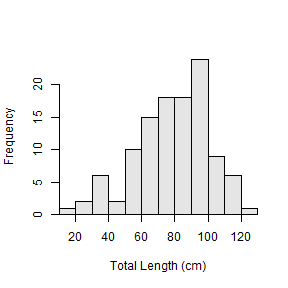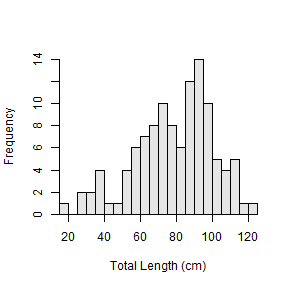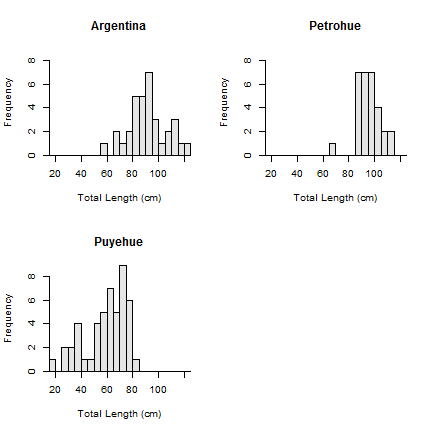## The Problem – Binning for Length Frequency Histograms

Fisheries scientists often make histograms of fish lengths. For example, the code below uses `hist()` (actually `hist.formula()`) from the `FSA` package to construct a histogram of total lengths for Chinook Salmon from Argentinian waters.The default bins for these histograms are rarely what the fisheries scientist desires. For example, the 10-cm wide bins shown above resulted in a histogram that lacked detail. Thus, the fisheries scientist may want to construct a histogram with 5-cm wide bins to reveal more detail.

As described in the Introductory Fisheries Analysis with R book, specific bin widths may be created by creating a sequence of numbers that represent the lower values of each bin. This sequence is most easily created with `seq()` which takes the minimum value, the maximum value, and a step value (which will be the bin width) as its three arguments. For example, the following constructs a histogram with 5-cm bin widths.Definining a sequence for bins is flexible, but it requires the user to identify the minimum and maximum value in the data. This is inefficient because it requires additional code or, more usually, constructing the plot once without any `breaks=`. In addition, the breaks are then “hard-wired” which de-generalizes the code and leads to more inefficiency.

As an example, imagine having a markdown template that will be used to construct a length frequency histogram for Chinook Salmon. Suppose that this template will be used to construct histograms for Chinook Salmon from different water bodies, years, etc. Chances are that you will always want 5-cm breaks for these histograms. However, with the hard-wired breaks described above, the user (you!) may have to change the first two values in `seq()` to reflect the minimum and maximum values for each current data.frame.1

## Solution – A Bin Width Argument for `hist.formula()`

To solve this problem, I have introduced the `w=` argument to `hist.formula()` in `FSA` that “smartly” sets the width of bins to be used in the histogram. The “smart” part is that the starting bin will be chosen based on the minimum observed value in the data and the value of `w=`. For example if the minimum observed value is 16 cm, then the starting bin will be 15 if `w=5`, 10 if `w=10`, and 0 if `w=25`.

For example, the same histogram constructed above with `seq()` is constructed below with `w=`.This works well in a template because you only need to decide what bin width you desire and don’t have to find the minimum and maximum values.

## Extension – Also Works with Muliple Histograms

The `w=` argument also works when multiple histograms are constructed.## Final Note

The `hist.formula()` with `w=` is currently in the development version of `FSA` package on GitHub (i.e., it is not available in v0.8.5 that is currently on CRAN). Please let me know what you think of this addition.

1. You could use the results from `min()` and `max()` in the `seq()`, but that is cumbersome code.